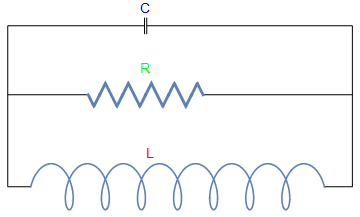# Preface

This section gives an introduction to modelling electric circuits.

Introduction to Linear Algebra with Mathematica

# Electric circuits

Example: Figure shows an electric circuit containing a capacitor C, a resistor R, and an inductor L, in parallel. Recall Kirchhoff's law:

• The sum of the currents flowing into any node of the system equals the sum of the currents flowing out of that node;
• The net voltage drops around each closed loop equals zero.
fbas[x_] := x/L /; 0 <= x < L
fbas[x_] := -(x - 2*L)/L /; L <= x < 3*L
fbas[x_] := (x - 4*L)/L /; 3*L <= x < 4*L
L = 1/2;
f[x_] := fbas[Mod[x, 4*L, -1/16^2*L]]
SetOptions[Plot, ImageSize -> 300];
resistor =
Plot[f[x], {x, -10*L, 10*L}, PlotRange -> {-1.2, 1.2},
PlotStyle -> Thickness[0.008], PlotLabel -> "R", Ticks -> None, Axes -> False]
coil = ParametricPlot[{1*Cos[t*3 + Pi] + 1.5*t - 9,
2*Sin[t*3] - 8}, {t, 0, 5*Pi}, PlotLabel -> "L", Ticks -> None, Axes -> False, ImageSize -> Tiny]
l1 = Graphics[Line[{{-0.1, 6.7}, {-0.1, 5.3}}]]
l2 = Graphics[Line[{{0.1, 6.7}, {0.1, 5.3}}]]
l3 = Graphics[Line[{{-10, -8}, {-12, -8}, {-12, 0}, {-5, 0}}]]
l4 = Graphics[Line[{{-12, 0}, {-12.0, 6}, {-0.1, 6}}]]
l5 = Graphics[Line[{{0.1, 6}, {18, 6}, {18, -8}, {15.5619, -8}}]]
l6 = Graphics[Line[{{5, 0}, {18, 0}}]]
textC = Graphics[Text[Style["C", FontSize -> 14, Blue], {0, 7.5}]]
textR = Graphics[Text[Style["R", FontSize -> 14, Green], {0, 2.2}]]
textL = Graphics[Text[Style["L", FontSize -> 14, Red], {0.5, -5.2}]]
Show[l1, l2, l3, l4, l5, l6, resistor, Graphics[coil, PlotRegion -> {{-1, 1}, {-1, 1}}], textR, textC, textL]